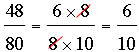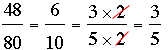SEARCH HOMEMath Central Quandaries & QueriesQuestion from Asia, a student: In a forest,it rained 36 out of 90 days. What is the decimal equivalent of 30 out of 90 daysHi Asia,

You say 36 days and then 30 days so I am not sure which you want. I'll show you how to do this with a slightly different question.

In a forest,it rained 48 out of 80 days. What is the decimal equivalent of 48 out of 80 days?

The fraction of days it rained is 48/80 so you need to convert this common fraction to a decimal fraction. I would first simplify the fraction. I know that 6 × 8 = 48 so 8 divides both the numerator and denominator. Hence.

But 2 divides the numerator and denominator of 6/10 so.

There are no more simplification of the fraction so all that remains is to convert 3/5 to a decimal fraction. To do so divide 5 into 3 using long division. I get 3/5 = 0.6 and hence 48 out of 80 days is 0.6.

I hope this helps,
PennyMath Central is supported by the University of Regina and The Pacific Institute for the Mathematical Sciences.